##### Econometrics# Introduction to Econometrics - Definitions and Properties of RandomVariables

 Many statistical models may be represented by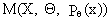(I.III-1) with the three elements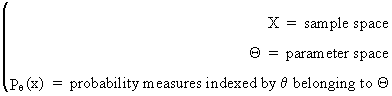(I.III-2) Sometimes it is possible to describe the probability measure in (I.III-2) by the probability density function (pdf)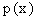(I.III-3) If a sample of observations is independent, identically distributed (iid) then the pdf can be written as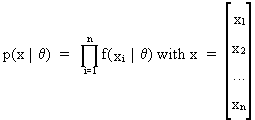(I.III-4) In Bayesian statistics an additional element is available. A prior pdf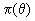(I.III-5) is introduced into the model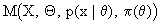(I.III-6) The prior pdf represents prior information about the possible parameter values (without using the observations).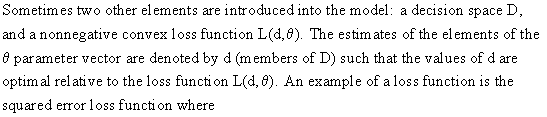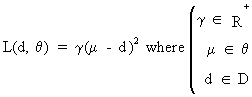(I.III-7)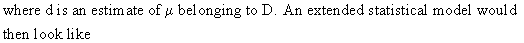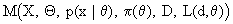(I.III-8) The aim of a lot of statistical research is to provide adequate theories and procedures in order to be able to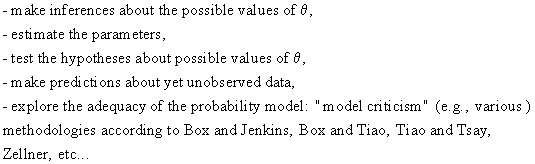A variable is called a random (c.q. stochastic) variable if the possible values of the variable have different probabilities. Therefore a random variable always has a probability density function (pdf) and a probability distribution. The relationship between distributions and probabilities can be defined as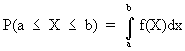(I.III-9) where f(X) is the probability density function. A cumulative probability distribution is defined as follows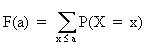(I.III-10) for discrete distributions, or as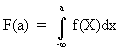(I.III-11) for continuous distributions. There are three properties of cumulative probability distributions (c.q. ogives):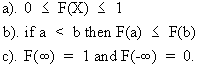Furthermore there is the very important notion of expectation that we 'll define now as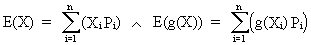(I.III-12) or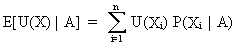(I.III-13) according to Jeffreys' definition. Both expressions (I.III-12) and (I.III-13) are formulated for discrete variables. Alternate definitions for continuous variables are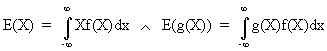(I.III-14) or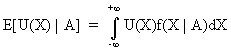(I.III-15) according to Jeffreys. Some very interesting properties of expectations have to be considered in order to provide us a sound mathematical basis for several proofs and theorems to be discussed in the following chapters: E(a X + b) = a E(X) + b where E(b) = b E(g(X) + h(X)) = E(g(X)) + E(h(X)) E(X + Y) = E(X) + E(Y) E(X Y) = E(X) E(Y) if, and only if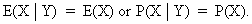(a and b are real numbers). The mathematical expectation can be thought of as the most probable value of a variable. The variance of a random variable is another very important property of probability distributions. It is in fact a measure for the spread of a stochastic variable. The easiest way to define a variance is by means of mathematical expectations as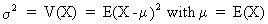(I.III-16) or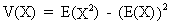(I.III-17) Equation (I.III-17) can be proved using (I.III-16) and the four properties of expectations as follows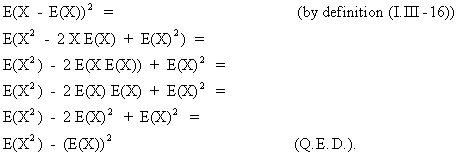Of course variances have properties similar to the properties of expectations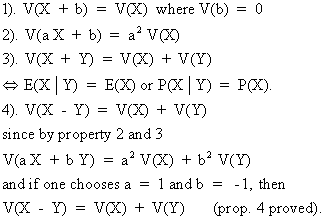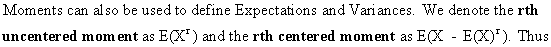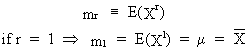(I.III-18) and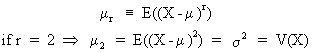(I.III-19) It can be shown that centered moments can quite easily be computed from uncentered moments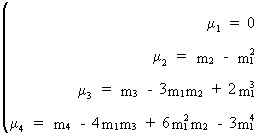(I.III-20) (see also eq. (I.III-17)). A probability distribution function can be characterized by its centered and uncentered moments (e.g., mean, variance, ...). Therefore a general method of deriving uncentered moments would be a very nice thing to have (the centered moments would then be computed from the conversion formulae (I.III-20)). This is not wishful thinking since the moment generating function of a discrete stochastic variable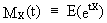(I.III-21)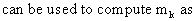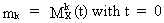(I.III-22)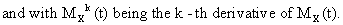Eq. (I.III-22) can be proved as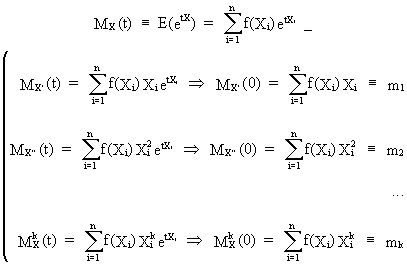(I.III-23) The covariance between two random variables can be defined easily using mathematical expectations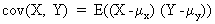(I.III-24) or equivalently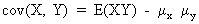(I.III-25) whereas the correlation is derived from the covariance as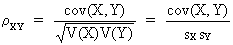(I.III-26)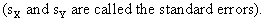The correlation between X and Y, as defined in, (I.III-26) lies between -1 and 1. Intuitively the covariance and the correlation can be thought of as a measure of collinearity of the points in a scatter plot (a plot of variable Y against the corresponding values of X). The only difference between covariance and correlation is that the latter has been standardized and is therefore independent of both variables' dimension. Above this, it is important to understand the following relationship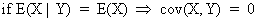(I.III-27) which clearly states that the implication is valid in one direction only, since the covariance and the correlation are by definition measures for linear relationships while the dependence of two random variables could by of any kind (linear and nonlinear)! So far nothing has been said about the probability distributions of the random variables. Theoretically of course there are an infinite number of possible probability density functions but only few of them are worthwhile discussing shortly because of their immense importance in econometrics and statistics. The binomial distribution can be defined as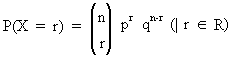(I.III-28) where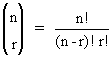(I.III-29) and p = chance of a success q = chance of a failure p + q = 1 n = number of independent draws X = number of successes. Furthermore E(X) = n p and V(X) = n p q. This can be proved by using the moment generating function (I.III-21) of (I.III-28) and applying (I.III-22) to it. The binomial distribution is of huge importance in quality control and experimental design. The most important probability distribution in statistics though is the normal distribution. This function is defined as follows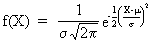(I.III-30) with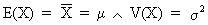(I.III-31) The mean and variance can be derived from the moment generating function. Above that, any normal distribution function is perfectly identified by its mean and variance. The fact that a random variable is normally distributed can be denoted as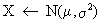(I.III-32) Having introduced this kind of notation for normal distributions, it is quite easy to describe the socalled additivity property.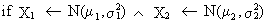(I.III-33) then, it follows that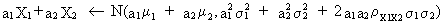(I.III-34) In other words, a linear function of normally distributed random variables is also normally distributed! Proof for the generalized additivity property for independent variables is straightforward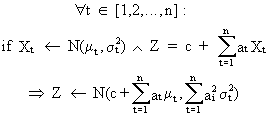(I.III-35) (for independent Xt variables) since on using the moment generating function we obtain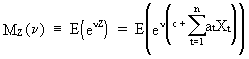(I.III-36)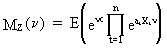(I.III-37)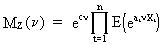(I.III-38)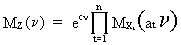(I.III-39)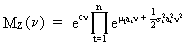(I.III-40) according to the explicit expression of the moment generating function of normal stochastic variables, yielding finally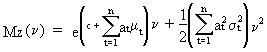(I.III-41) which proves the property (Q.E.D.). Analogously a generalized additivity property for dependent variables can be obtained (see eq. (I.III-33) and (I.III-34)). This is however not necessary in our further discussions and thus beyond the scope of this work. Derived from the normal distribution is the log-normal distribution. A random variable is log-normally distributed if, and only if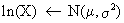(I.III-42) and if (by definition of ln)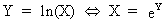(I.III-43) The expectation and variance of this probability density function is given by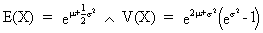(I.III-44) As previously stated a probability distribution function of a random variable can be formulated using probability theory, e.g., in (I.III-9) and (I.III-11). It is however imperative to formalize another link between probability and distribution theory: the Bienaymé-Chebyshev theorem (or inequality).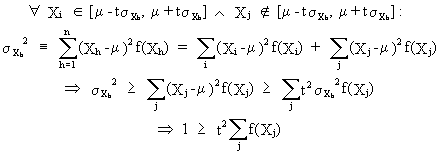(I.III-45) Since we defined in (I.III-45)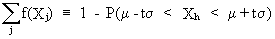(I.III-46) it is obvious that, on combining (I.III-45) and (I.III-46), the following holds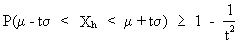(I.III-47) which is the Bienaymé-Chebyshev inequality.© 2000-2022 All rights reserved. All Photographs (jpg files) are the property of Corel Corporation, Microsoft and their licensors. We acquired a non-transferable license to use these pictures in this website.
The free use of the scientific content in this website is granted for non commercial use only. In any case, the source (url) should always be clearly displayed. Under no circumstances are you allowed to reproduce, copy or redistribute the design, layout, or any content of this website (for commercial use) including any materials contained herein without the express written permission.

Information provided on this web site is provided "AS IS" without warranty of any kind, either express or implied, including, without limitation, warranties of merchantability, fitness for a particular purpose, and noninfringement. We use reasonable efforts to include accurate and timely information and periodically updates the information without notice. However, we make no warranties or representations as to the accuracy or completeness of such information, and it assumes no liability or responsibility for errors or omissions in the content of this web site. Your use of this web site is AT YOUR OWN RISK. Under no circumstances and under no legal theory shall we be liable to you or any other person for any direct, indirect, special, incidental, exemplary, or consequential damages arising from your access to, or use of, this web site.

Contributions and Scientific Research: Prof. Dr. E. Borghers, Prof. Dr. P. Wessa
Please, cite this website when used in publications: Xycoon (or Authors), Statistics - Econometrics - Forecasting (Title), Office for Research Development and Education (Publisher), http://www.xycoon.com/ (URL), (access or printout date).